# Title 40

## SECTION 1066.630

### 1066.630 PDP, SSV, and CFV flow rate calculations.

§ 1066.630 PDP, SSV, and CFV flow rate calculations.

This section describes the equations for calculating flow rates from various flow meters. After you calibrate a flow meter according to § 1066.625, use the calculations described in this section to calculate flow during an emission test. Calculate flow according to 40 CFR 1065.642 instead if you calculate emissions based on molar flow rates.

(a) PDP. (1) Based on the speed at which you operate the PDP for a test interval, select the corresponding slope, a1, and intercept, a0, as determined in § 1066.625(a), to calculate PDP flow rate, v , as follows: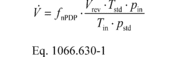Where: fnPDP = pump speed. Vrev = PDP volume pumped per revolution, as determined in paragraph (a)(2) of this section. Tstd = standard temperature = 293.15 K. pin = static absolute pressure at the PDP inlet. Tin = absolute temperature at the PDP inlet. pstd = standard pressure = 101.325 kPa.

(2) Calculate Vrev using the following equation: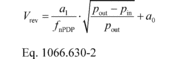pout = static absolute pressure at the PDP outlet.

Example: a1 = 0.8405 m 3/s fnPDP = 12.58 r/s pout = 99.950 kPa pin = 98.575 kPa a0 = 0.056 m 3/r Tin = 323.5 K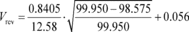Vrev = 0.063 m 3/r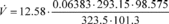v = 0.7079 m 3/s

(b) SSV. Calculate SSV flow rate, v , as follows: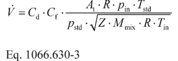Where: Cd = discharge coefficient, as determined based on the Cd versus Re# equation in § 1066.625(b)(2)(viii). Cf = flow coefficient, as determined in § 1066.625(b)(2)(ii). At = venturi throat cross-sectional area. R = molar gas constant. pin = static absolute pressure at the venturi inlet. Tstd = standard temperature. pstd = standard pressure. Z = compressibility factor. Mmix = molar mass of gas mixture. Tin = absolute temperature at the venturi inlet. Example: Cd = 0.890 Cf = 0.472 At = 0.01824 m 2 R = 8.314472 J/(mol·K) = 8.314472 (m 2·kg)/(s 2·mol·K) pin = 98.496 kPa Tstd = 293.15 K pstd = 101.325 kPa Z = 1 Mmix = 28.7789 g/mol = 0.0287789 kg/mol Tin = 296.85 K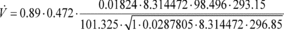V = 2.155 m 3/s

(c) CFV. If you use multiple venturis and you calibrated each venturi independently to determine a separate calibration coefficient, Kv, for each venturi, calculate the individual volume flow rates through each venturi and sum all their flow rates to determine CFV flow rate, V . If you use multiple venturis and you calibrated venturis in combination, calculate V using the Kv that was determined for that combination of venturis.

(1) To calculate V through one venturi or a combination of venturis, use the mean Kv you determined in § 1066.625(c) and calculate V as follows: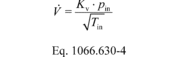Where: Kv = flow meter calibration coefficient. Tin = temperature at the venturi inlet. pin = absolute static pressure at the venturi inlet. Example: Kv = 0.074954 m 3·K 0.5/(kPa·s) pin = 99.654 kPa Tin = 353.15 K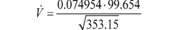V = 0.39748 m 3/s

(2) [Reserved]

[81 FR 74211, Oct. 25, 2016]